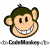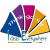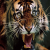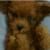## 【面试题】10000个数字求第二大的数字，不许用排序算法 热

10000个数字求第二大的数字，不许用排序算法

1
d

```import java.util.*;

public class Compare{

public static void main(String[] args) {
int[] intArray=new int;
Random r=new Random();
for(int i=0;i<intArray.length;i++){
intArray[i]=r.nextInt(10000);
System.out.print(intArray[i]+"   ");
}

int maxNum=intArray;
int secMaxNum=0;
int j=1;
while(j<intArray.length){
int temp=intArray[j];
if(maxNum>temp){
if(temp>secMaxNum){
secMaxNum=temp;
}
}else{
secMaxNum=maxNum;
maxNum=temp;
}

j++;
}
Arrays.sort(intArray);
for(int c:intArray){
System.out.println(c+" ");
}

System.out.println(maxNum+" "+secMaxNum);
}
}```
d

1```public static void max2rd(int len) {
int[] arr = new int[len];
int[] max = new int;
for(int i = 0; i < len; arr[i ++] = new Random().nextInt(1777));
for(int num : arr) {
System.out.print(num + ",");
if(num > max && num < max) max = num;
if(num > max) {
int tmp = max;
max = num;
max = tmp;
}
}
System.out.println();
System.out.println("Max1st = " + max);
System.out.println("Max2rd = " + max);
}```

1max1=0;//存最大
max2=0; //存次大；仅是算法，暂考虑非负值。
if(x>max1){
max2=max1;max1=x;
}elseif(max1>x and x>max2){
max2=x;
}
0

0
s

0int max=arr;//表示为最大
int maxindex=0;//表示最大的索引
int ded=arr;
for(int i=1;i<arr.length;i++){
if(arr[i]>max){
maxindex=i;
max=arr[i];
}
}
//第一遍找到最大的数

//第二遍找第二大的数，如果遇到最大的索引就略过
for(int i=1;i<arr.length;i++){
if(i==maxindex)
continue;
if(arr[i]>ded)
ded=arr[i];

}
0
s

0```var a = [];//10000个数字
var m1 = 0,m2 = 0;
a.forEach(function(val, index){
if(val > m1){
if(m1 > m2) m2 = m1;
m1 = val;
}else if(val > m2){
m2 = val;
}
})
console.log(m1, m2);```m1=a;m2=a; 是不是好些
T
@hmh 题目没有说数字全部大于0
T